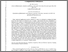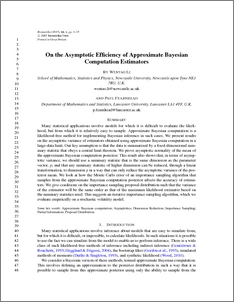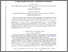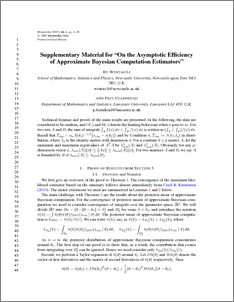# On the asymptotic efficiency of approximate Bayesian computation estimators

Li, Wentao and Fearnhead, Paul (2018) On the asymptotic efficiency of approximate Bayesian computation estimators. Biometrika, 105 (2). pp. 285-299. ISSN 0006-3444Preview
PDF (ABCasymptotics)
ABCasymptotics.pdf - Accepted VersionPreview
PDF (Supplementary Material)
Supplement.pdf - Accepted Version

## Abstract

Many statistical applications involve models for which it is difficult to evaluate the likelihood, but from which it is relatively easy to sample. Approximate Bayesian computation is a likelihood-free method for implementing Bayesian inference in such cases. We present results on the asymptotic variance of estimators obtained using approximate Bayesian computation in a large-data limit. Our key assumption is that the data is summarized by a fixed-dimensional summary statistic that obeys a central limit theorem. We prove asymptotic normality of the mean of the approximate Bayesian computation posterior. This result also shows that, in terms of asymptotic variance, we should use a summary statistic that is the same dimension as the parameter vector, p; and that any summary statistic of higher dimension can be reduced, through a linear transformation, to dimension p in a way that can only reduce the asymptotic variance of the posterior mean. We look at how the Monte Carlo error of an importance sampling algorithm that samples from the approximate Bayesian computation posterior affects the accuracy of estimators. We give conditions on the importance sampling proposal distribution such that the variance of the estimator will be the same order as that of the maximum likelihood estimator based on the summary statistics used. This suggests an iterative importance sampling algorithm, which we evaluate empirically on a stochastic volatility model.

Item Type:
Journal Article
Journal or Publication Title:
Biometrika
This is a pre-copy-editing, author-produced PDF of an article accepted for publication in Biometrika following peer review. The definitive publisher-authenticated version Wentao Li, Paul Fearnhead; On the asymptotic efficiency of approximate Bayesian computation estimators, Biometrika, Volume 105, Issue 2, 1 June 2018, Pages 285–299, https://doi.org/10.1093/biomet/asx078 is available online at: https://academic.oup.com/biomet/article/105/2/285/4818354
Uncontrolled Keywords:
/dk/atira/pure/subjectarea/asjc/1100/1101
Subjects:
?? APPROXIMATE BAYESIAN COMPUTATIONASYMPTOTICSDIMENSION REDUCTION IMPORTANCE SAMPLINGPARTIAL INFORMATIONPROPOSAL DISTRIBUTIONAGRICULTURAL AND BIOLOGICAL SCIENCES(ALL)APPLIED MATHEMATICSSTATISTICS AND PROBABILITYSTATISTICS, PROBABILITY AND UNCERTAINTYMATHEMAT ??
Departments:
ID Code:
74491
Deposited By:
Deposited On:
03 Jul 2015 13:42
Refereed?:
Yes
Published?:
Published